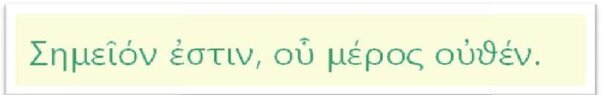# What the result of null added to itself?

• B
somok
TL;DR Summary
The null part added to itself will always remain the same as itself, namely null.
Hey,...

is that correct to say that "The null part added to itself will always remain the same as itself, namely null.""

In arithmetic, 3 * 0 = 0 + 0 + 0 = 0;

It is therefore healthy to extrapolate as follows:

∞-1 * 0 = 0 + 0 + 0 +... + 0 + 0 + 0 + 0 = 0
∞ * 0 = 0 + 0 + 0 +... + 0 + 0 + 0 + 0 + 0 =0

Last edited:

Homework Helper
Gold Member
2022 Award
There is no number called "infinite".

somok
you are right ... it is a mistake from me...

take ∞ for account

Infinity, denoted ∞, is not a number, but a concept or a phenomenon. We can, for example, say that the value of a variable x grows positively by taking larger and larger values; we will then say that x tends to infinity.

Mayhem
you are right ... it is a mistake from me...

take ∞ for account

Infinity, denoted ∞, is not a number, but a concept or a phenomenon. We can, for example, say that the value of a variable x grows positively by taking larger and larger values; we will then say that x tends to infinity.
Perhaps formalize this a bit more clearly with limits.

somok
Perhaps formalize this a bit more clearly with limits.

@Mayhem

stay focus to my initial question...pls

is that correct to say that :

"" The null part added to itself will always remain the same as itself, namely null.""

think about geometry if you have more facilities :Translation: A point is that which has no part.
(Euclid's Elements)

Euclid defines it as "that whose part is zero", that is, a dot has no width, length or height

Mentor
2022 Award
. while A=0
. A=A * 0

does not end, yes. However, this does not allow you to write ##\infty \cdot 0=0## because ##\infty \cdot## is simply not defined. It is like discussing the shoe size of Big Foot - meaningless.

somok
. while A=0
. A=A * 0

does not end, yes. However, this does not allow you to write ##\infty \cdot 0=0## because ##\infty \cdot## is simply not defined. It is like discussing the shoe size of Big Foot - meaningless.

for sure

if A=0 so 0=0*0

it s not what I'm saying...pls...

here is what i say...

3 * 0 = 0 + 0 + 0 = 0;

•PeroK
somok
think about geometry if you have more facilities :

View attachment 302718

Translation: A point is that which has no part.
(Euclid's Elements)

Euclid defines it as "that whose part is zero", that is, a dot has no width, length or height

Mentor
2022 Award
here is what i say...

3 * 0 = 0 + 0 + 0 = 0;

Well, we cannot fill our pages with trivia.

•